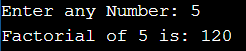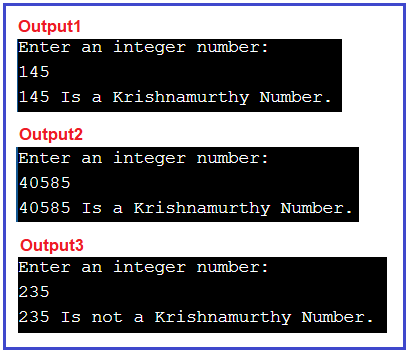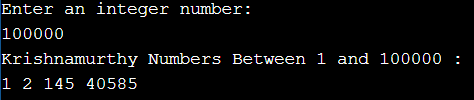# Krishnamurthy Number in C#

## Krishnamurthy Number in C# with Examples

In this article, I am going to discuss How to Implement Krishnamurthy Number Program in C# with Examples. Please read our previous article where we discussed the Kaprekar Number Program in C#. Here, in this article, first, we will learn what is a Krishnamurthy Number and then we will see how to implement the Krishnamurthy Number Program in C#. And finally, we will see how to print all the Krishnamurthy numbers between a range of numbers like between 1 to 100 or 100 to 1000, etc.

##### What is a Krishnamurthy Number?

A Krishnamurthy number is a number whose sum of the factorial of each digit is equal to the number itself. For example, 145, sum of factorial of each digit:  1! + 4! + 5! = 1 + 24 + 120 = 145.

Examples:
Input: 145
Output: YES
Explanation: 1! + 4! + 5! = 1 + 24 + 120 = 145, which is equal to the input number itself, hence it is a Krishnamurthy Number.

Input: 235
Output: NO
Explanation: 2! + 3! + 5! = 2 + 6 + 120 = 128, which is not equal to the input number, and hence it is not a Krishnamurthy Number.

First of all, we have to know what is Factorial number. how does it work? What is the basic method to solve this problem?
Factorial number: Factorial of n is the product of all positive descending integers.
For example
1) 4! = 4*3*2*1 = 24
2) 3! = 3*2*1 = 6

###### Factorial of a number in C#
```using System;
public class FactorialNumber
{
public static void Main ()
{
int fact = 1;
Console.Write ("Enter any Number: ");
for (int i = 1; i <= number; i++)
{
fact = fact * i;
}
Console.Write ("Factorial of " + number + " is: " + fact);
}
}
```
###### Output:After understanding how to calculate the factorial of a number, let’s come to our main question which is the Krishnamurthy number.

##### How to implement Krishnamurthy Number Program in C#?

We are going to use the very basic method to implement this program but before that, you should have some basic knowledge of the Divide operator. The idea is very simple, we need to compute the sum of factorials of each digit of the input number and then compare the sum with the input number.  Here are some steps to follow: –

1. Using one temporary variable temp, store a copy of the original number.
2. Initialize the variable sum with zero. It will store the sum of the factorial of each individual digit.
3. Use loop until temp is not equal to zero,
4. Get the last digit of variable temp, and store it in the variable lastDigit.
5. Calculate the factorial of variable lastDigit.
6. Add the factorial result into the variable sum.
7. Remove the last digit of the number.
8. Compare the original number with the value of the variable sum. If both are equal then the number is Krishnamurthy Number, else it is not.
##### Example: Krishnamurthy Number Program in C#

The following sample code shows how to implement the Krishnamurthy number program in C#.

```using System;
public class KrishnamurthyNumber
{
// method to Check Krishnamurthy number
public static bool IsKrishnamurthy (int number)
{
//Declare Variables
int sum = 0, lastDigit = 0;
int tempNum = number;

// traverse through all digits of number
while (tempNum != 0)
{
lastDigit = tempNum % 10;
sum += Convert.ToInt32(Factorial(lastDigit));
tempNum /= 10;
}

// compare sum and number
if (sum == number)
return true;
return false;
}

//Method to calculate factorial of an integer
public static long Factorial (int number)
{
long fact = 1;
for (int i = 1; i <= number; i++)
{
fact *= i;
}
return fact;
}

//Main method
public static void Main ()
{
//Take input from end-user
Console.WriteLine ("Enter an integer number:");
int number = Convert.ToInt32 (Console.ReadLine ());

// check number is Krishnamurthy number
bool result = IsKrishnamurthy(number);
if(result)
Console.WriteLine(number + " Is a Krishnamurthy Number.");
else
Console.WriteLine(number + " Is not a Krishnamurthy Number.");

}
}
```
###### Output:Note: Sometimes the Krishnamurthy number is also called Strong number, Special number, and Peterson number.

##### C# Program to Print all Krishnamurthy Numbers Between 1 and N

The following C# Program will allow the user to input a number. Then it will print all the Krishnamurthy numbers between 1 and that input number.

```using System;
public class KrishnamurthyNumber
{
// method to Check Krishnamurthy number
public static bool IsKrishnamurthy (int number)
{
//Declare Variables
int sum = 0, lastDigit = 0;
int tempNum = number;

// traverse through all digits of number
while (tempNum != 0)
{
lastDigit = tempNum % 10;
sum += Convert.ToInt32(Factorial(lastDigit));
tempNum /= 10;
}

// compare sum and number
if (sum == number)
return true;
return false;
}

//Method to calculate factorial of an integer
public static long Factorial (int number)
{
long fact = 1;
for (int i = 1; i <= number; i++)
{
fact *= i;
}
return fact;
}

//Main method
public static void Main ()
{
//Take input from end-user
Console.WriteLine ("Enter an integer number:");
int number = Convert.ToInt32 (Console.ReadLine ());

Console.WriteLine("Krishnamurthy Numbers Between 1 and " + number + " : ");
for (int i = 1; i <= number; i++)
{
bool result = IsKrishnamurthy(i);
if(result)
Console.Write(i + " ");
}